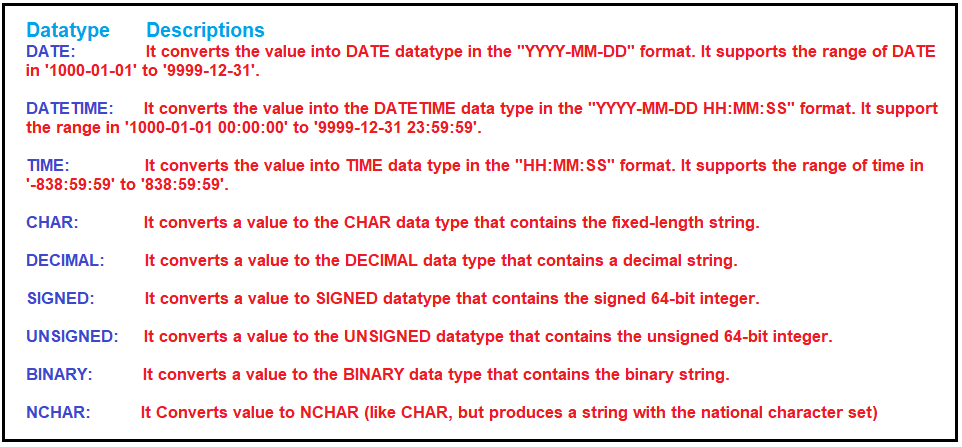# MySQL CAST Function

## MySQL CAST Function with Examples

In this article, I am going to discuss MySQL CAST Function with Examples. Please read our previous article where we discussed MySQL COALESCE Function with Examples.

##### MySQL CAST Function

The MySQL CAST function is used to convert a value or expression from one data type to another data type. The changing of one data type to another data type is known as casting. The casting is very useful while importing or exporting the data. It is mostly used with WHERE, HAVING, and JOIN clauses. This function is similar to the CONVERT function in MySQL which we will discuss in our next article.

###### Syntax:

Following is the syntax to use the CAST function. The MySQL CAST function accepts two parameters as shown in the below image.###### Parameters
1. Expression: Required: This is the value that needs to be converted.
2. Datatype: Required: It is used to specify the data type in which the value needs to be converted.

Return Value: The CAST function returns a value in the desired datatype after conversion.

following are the datatypes to which the CAST function works perfectly:##### Examples:

Example: Converting a value to DATE datatype using the CAST function.
SELECT CAST(“2020-10-25” AS DATE) AS CastDate;
Output: 2020-10-25

Example: Converting a value to DATETIME datatype using the CAST function.
SELECT CAST(“2020-10-25” AS DATETIME) AS CastDateTime;
Output: 2020-10-25 00:00:00

Example: Converting a value to TIME datatype using the CAST function.
SELECT CAST(“2020-10-25 08:14:57” AS TIME) AS CastTime;
Output: 08:14:57

Example: Converting a value to CHAR datatype using the CAST function.
SELECT CAST(‘123’ AS CHAR) AS CastChar;
Output: 123

Example: Converting a value to SIGNED datatype using the CAST function.
SELECT CAST(20 – 44 AS SIGNED) AS CastSigned;
Output: -24

Example: Converting a value to UNSIGNED datatype using the CAST function.
SELECT CAST(20 – 44 AS UNSIGNED) AS CastUnsigned;
Output: 18446744073709551592

Example: Converting a value to DECIMAL datatype using the CAST function.
SELECT CAST(‘4.4’ AS DECIMAL(6,2)) AS CastDecimal;
Output: 4.40